## Laplace's Equation

The scalar form of Laplace's equation is the Partial Differential Equation(1)

It is a special case of the Helmholtz Differential Equation(2)

with, or Poisson's Equation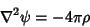(3)

with. The vector Laplace's equation is given by(4)

A Functionwhich satisfies Laplace's equation is said to be Harmonic. A solution to Laplace's equation has the property that the average value over a spherical surface is equal to the value at the center of the Sphere (Gauss's Harmonic Function Theorem). Solutions have no local maxima or minima. Because Laplace's equation is linear, the superposition of any two solutions is also a solution.

A solution to Laplace's equation is uniquely determined if (1) the value of the function is specified on all boundaries (Dirichlet Boundary Conditions) or (2) the normal derivative of the function is specified on all boundaries (Neumann Boundary Conditions).

Laplace's equation can be solved by Separation of Variables in all 11 coordinate systems that the Helmholtz Differential Equation can. In addition, separation can be achieved by introducing a multiplicative factor in two additional coordinate systems. The separated form is(5)

and setting(6)

where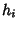are Scale Factors, gives the Laplace's equation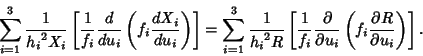(7)

If the right side is equal to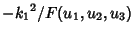, whereis a constant and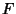is any function, and if(8)

whereis the Stäckel Determinant, then the equation can be solved using the methods of the Helmholtz Differential Equation. The two systems where this is the case are Bispherical and Toroidal, bringing the total number of separable systems for Laplace's equation to 13 (Morse and Feshbach 1953, pp. 665-666).

In 2-D Bipolar Coordinates, Laplace's equation is separable, although the Helmholtz Differential Equation is not.

See also Boundary Conditions, Harmonic Equation, Helmholtz Differential Equation, Partial Differential Equation, Poisson's Equation, Separation of Variables, Stäckel Determinant

References

Abramowitz, M. and Stegun, C. A. (Eds.). Handbook of Mathematical Functions with Formulas, Graphs, and Mathematical Tables, 9th printing. New York: Dover, p. 17, 1972.

Morse, P. M. and Feshbach, H. Methods of Theoretical Physics, Part I. New York: McGraw-Hill, pp. 125-126, 1953.